# Weil-Châtelet group

(Redirected from Weil–Châtelet group)
The group of principal homogeneous spaces (cf. Principal homogeneous space) over an Abelian variety. It was shown by A. Weil  and, in one special case, by F. Châtelet, that for an arbitrary Abelian varietyover a field, the setof principal homogeneous spaces over, defined over k, has a group structure. The group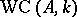is isomorphic to the first Galois cohomology group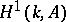. The groupis always periodic; moreover, if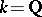, it contains elements of arbitrary orders , . According to Lang's theorem,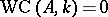ifis a finite field. The index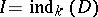, equal to the smallest degree of an extensionfor which there exists a-rational point, is defined for any element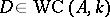. Ifandis an algebraic function field over an algebraically closed field of constants or a local field,becomes identical with the order ofin the group, . In the general case these numbers are different, but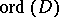is always a divisor of. The grouphas been computed for local fields(see, for instance, , , ).
Ifis a global field, the computation of the groupis based on the reduction homomorphismswhereis an arbitrary valuation ofand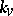is the completion ofwith respect to. The kernel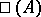of the homomorphism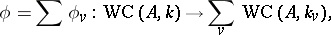known as the Tate–Shafarevich group of the Abelian variety, has been computed only in the case whenis a field of algebraic functions of one variable over an algebraically closed field of constants , , . The co-kernel ofhas also been described in this case (up to the-component, whereis the characteristic of). The results of these calculations are used in the theory of elliptic surfaces. Ifis an algebraic number field, the structure of the grouphas not been studied to any great extent.